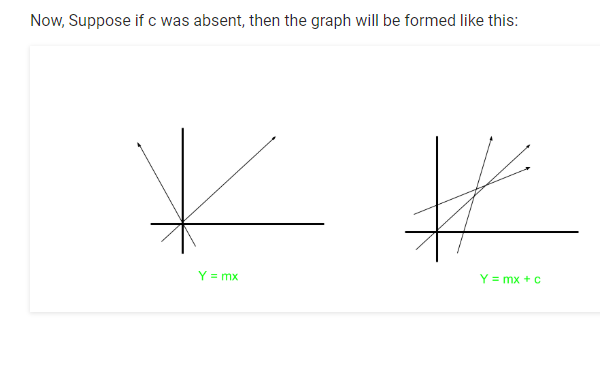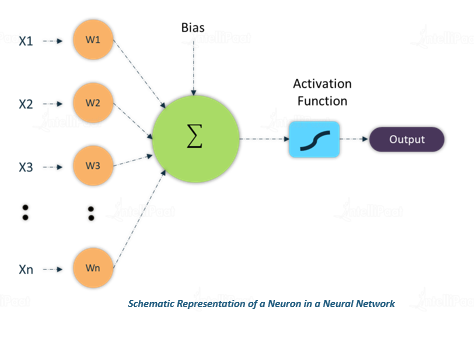+1 vote
3 views
I know about the Gradient Descent & Back-propagation Theorem. What I didn't get is: When and how to use Bias?

Ex - When mapping the AND function, when I use 2 inputs and 1 output, it does not give the correct weights, however, when I use 3 inputs (1 of which is a bias), it gives the correct weights.

by (10.9k points)

Bias is just like an intercept added in a linear equation. It is an additional parameter in the Neural Network which is used to adjust the output along with the weighted sum of the inputs to the neuron. Moreover, bias value allows you to shift the activation function to either right or left.

output  =  sum (weights * inputs) + bias

The output is calculated by multiplying the inputs with their weights and then passing it through an activation function like the Sigmoid function, etc. Here, bias acts like a constant which helps the model to fit the given data. The steepness of the Sigmoid depends on the weight of the inputs.

A simpler way to understand bias is through a constant c of a linear function

y =mx + c

It allows you to move the line down and up fitting the prediction with the data better. If the constant c is absent then the line will pass through the origin (0, 0) and you will get a poorer fit.

Neural Network is an important component of Machine Learning. You can go through this Machine Learning Course and become a Machine Learning expert.

by (108k points)
edited by

In a Neural network, weight increases the steepness of activation function and it decides how fast the activation function will trigger whereas bias is used to delay the triggering of the activation function.

For a typical neuron model, if the inputs are a1,a2,a3, then the weight applied to them are denoted as h1,h2,h3. Then the output is as follows:

y  = f(x)  = Σaihi

Where i is the number of inputs.

This shows us the effectiveness of a particular input. The weight of input is directly proportional to the impact of the network.

Bias is like the intercept added in a linear equation. It is an additional parameter in the Neural Network which is used to adjust the output along with the weighted sum of the inputs to the neuron. Thus, Bias is a constant which helps the model in a way that it can fit best for the given data.

The processing done by the neuron is:

output  = sum (weights * inputs) + bias

For example, consider an equation y=mx+c

Here m is acting as weight and the constant c is acting as bias.Due to the absence of bias, the model will train over point passing through origin only, which is not in accordance with a real-world scenario. Also with the introduction of bias, the model will become more flexible.

It helps the model to learn the patterns and it acts like an input node that always produces constant value 1 or other constant. Because of this property, they are not connected to the previous layer.

Bias units are not connected to any previous layer, it is just appended to the start/end of the input and each hidden layer, and is not affected by the values in the previous layer.

You can refer to this diagram:Here x1,x2,x3 are inputs and w1, w2,w3 are weights. It takes an input, processes it, passes it through an activation function, and returns the output.

Watch this video to learn about Neural Networks: